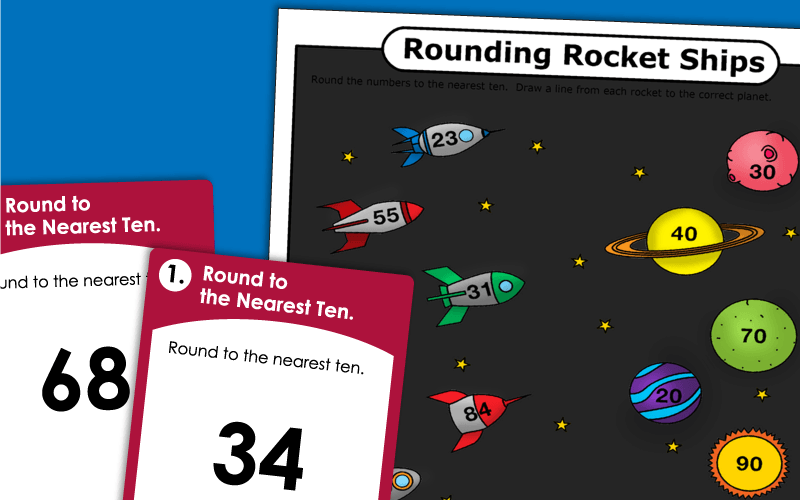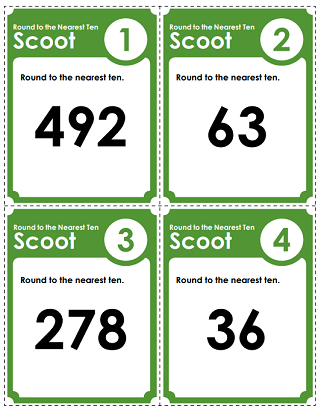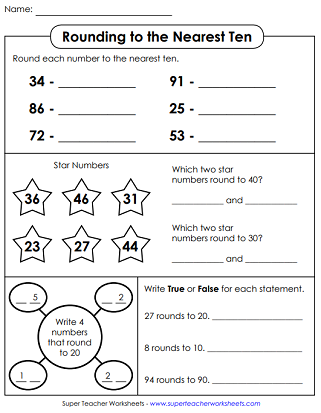# Rounding Numbers (Nearest Ten)

Round 2 and 3-digit numbers to the Nearest Ten. This page includes rounding worksheets, a Scoot game, task cards, and rounding tables.## Nearest Ten2-Digit Numbers

Use the numbers lines to round each number to the nearest 10.
Part 1: Round double-digit numbers to the nearest ten. Part 2: Star numbers. Part 3: Write four numbers on the web that round to 20. Part 4: True and False.
Round up and down for each number. Circle the number that is rounded to the nearest ten. Includes only two-digit numbers.
These task cards have 2-digit numbers that students can round to the nearest ten. Use these cards for class scavenger hunts, or as math learning centers.
Round the number to the nearest ten, then tell whether you rounded up or rounded down.
Cut out the numbers at the bottom of the page. Round each number and glue the answer next to each given number.
In this whole-class game, students travel around the classroom to different task cards. Each card has a number on it. Students round the number to the nearest ten.
Help the rockets land on the correct planets by rounding the 2-digit numbers to the nearest 10.
Decode the secret number symbols. Then round each number. Includes only single and double-digit numbers.
Round to the nearest ten to complete each bubble in the rounding caterpillar.
This worksheet has 22 double-digit numbers for students to round. The last two are cupcake-themed word problems.

## Nearest Ten(2 and 3-Digit Numbers)

Use the number line to learn how to round to the nearest ten.
Round these 3-digit numbers to the nearest ten.  (example:  465 rounds to 470)
Why did Humpty Dumpty have a great fall? To solve the math riddle, round each of the given numbers to the nearest ten. (Includes 2 and 3-digit numbers.)
In this game, students will move from desk to desk, finding numbers and rounding them to the nearest ten.
Print out and cut apart 30 task cards. Each one has a 2 or 3-digit number. Round each to the nearest ten. Use for math games, class scavenger hunts, and learning centers.
First, use the cipher code key at the top of the page to translate the picture symbols into regular numbers. Then round each number to the nearest ten. Includes 2 and 3-digit numbers.
Students begin by making a foldable, origami fortune teller (cootie catcher) game. When they play, students will round 2-digit numbers to the nearest ten.

## Rounding Charts(Nearest Ten)

This hundreds chart has arrows to help students determine whether to round up or round down.
This color chart uses a color-code to help students learn rounding.

## Nearest Tens & HundredsMixed

Use the number lines shown to round each number to the nearest ten or the nearest hundred.
Round each number to the nearest ten.  Then round to the nearest hundred.
A set of questions for rounding to the nearest ten and hundred.
Answer these word problems and questions on rounding numbers.
In this file, you'll find thirty task cards for rounding to the nearest ten and the nearest hundred. Use these cards on your document camera, for learning centers, or classroom scavenger hunts.
Rounding (Nearest Hundred)

This page has a collection of worksheets, number charts, and activities for rounding to the nearest hundred.

## Rounding Worksheet ImagesMy Account
Site Information